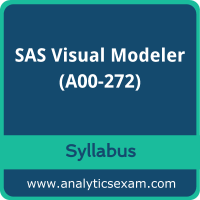# SAS A00-272 Certification Exam SyllabusThis page is a one-stop solution for any information you may require for SAS Certified Visual Modeler Using SAS Visual Statistics 7.4 (A00-272) Certification exam. The SAS A00-272 Exam Summary, Syllabus Topics and Sample Questions provide the base for the actual SAS Interactive Model Building and Exploration Using SAS Visual Statistics 7.4 exam preparation, we have designed these resources to help you get ready to take your dream exam.

The SAS Certified Visual Modeler Using SAS Visual Statistics 7.4 credential is globally recognized for validating SAS Visual Modeler knowledge. With the SAS Interactive Model Building and Exploration Using SAS Visual Statistics 7.4 Certification credential, you stand out in a crowd and prove that you have the SAS Visual Modeler knowledge to make a difference within your organization. The SAS Certified Visual Modeler Using SAS Visual Statistics 7.4 Certification (A00-272) exam will test the candidate's knowledge on following areas.

## SAS A00-272 Exam Summary:

 Exam Name SAS Certified Visual Modeler Using SAS Visual Statistics 7.4 Exam Code A00-272 Exam Duration 90 minutes Exam Questions 50 Multiple choice questions Passing Score 68% Exam Price \$180 (USD) Training SAS® Visual Statistics: Interactive Model Building Exam Registration Pearson VUE Sample Questions SAS Visual Modeler Certification Sample Question Practice Exam SAS Visual Modeler Certification Practice Exam

## SAS A00-272 Exam Topics:

Objective Details

## SAS® Visual Statistics Cross-functional Tasks – 18%

Prepare data using SAS® Visual Analytics. - Manage explorations and visualizations.
- Impute a variable.
- Transform a variable.
- Create an aggregated measure.
- Replace dirty data with missing values.
- Combine multiple categories into fewer levels.
- Create dummy variables in SAS® Visual Analytics and SAS® Visual Data Builder.
Filter data used for a model. - Exclude selections to filter data.
- Apply filters to visualization and data source.
- Review Measure Details.
Use interactive group-by. - Explain group-by modeling.
- Assign a group-by variable to a predictive model (logistic regression, linear regression model and generalized linear model).
- Interactively examine the Fit Summary for group-by models.
- Choose the best fitting group-by model using fit statistics and Variable Importance.
- Interpret model results using advanced group-by feature.
- Examine the summary table for group-by processing.

## Building and Assessing Segmentation Models – 32%

Perform unsupervised segmentation using cluster analysis. - Explain unsupervised classification.
- Given a scenario, set proper inputs for k-means algorithm.
- Build a cluster analysis in SAS® Visual Statistics.
- Assign roles for cluster analysis.
- View and edit cluster properties.
- Set Parallel Coordinate properties for a cluster.
- Given a scenario, appropriately change the number of clusters.
- Derive a cluster ID variable and use it in another visualization.
Analyze cluster results. - Interpret a Cluster Matrix.
- Interpret Parallel Coordinates plot.
- Interpret Cluster Summary tab.
Perform supervised segmentation using decision trees. - Explain how split points are determined.
- Assign variable roles for a decision tree.
- Define decision tree properties.
- Describe how predictions are formulated for a decision tree.
- Explain variable selection methods for decision trees.
- Derive a leaf ID for use in other models.
- Prune a decision tree.
Asses decision tree results. - Interpret tree with Tree Map.
- Interpret Leaf statistics.
- Interpret Assessment panel.
- Investigate leaf nodes.
- Explain icicle plot.

## Building and Assessing Regression-type Models – 40%

Explain linear models. - Explain linear regression.
- Model effects usage.
- Given a scenario, determine when to use a linear regression model vs. a generalized linear model.
Perform linear regression modeling. - Assign linear regression roles.
- Define linear regression properties.
- Explain informative missingness.
- Review outlier details and exclude outliers.
Perform generalized linear regression modeling. - Assign generalized linear model roles.
- Assign offset variable.
- Define linear regression properties.
- Link functions and distributions in generalized linear models.
- Given a scenario, choose appropriate distribution and link function.
Perform logistic regression modeling. - Explain logistic regression essentials.
- Explain prediction in logistic regression.
- Explain variable selection in SAS® Visual Statistics.
- Specify which variable is the event (binary).
- Specify how a multinomial response variable is used in SAS® Visual Statistics.
- Assign logistic regression roles.
- Define logistic regression properties.
- Specify when to use appropriate link function when building a predictive model.
Assess model results. - Interpret Fit Summary window.
- Interpret Residual Plot.
- Interpret ROC chart (KS Statistic).
- Evaluate Misclassification plot.
- Evaluate the Lift chart.
- Interpret Influence plot.
- Interpret Summary bar.
- Assess residuals and other model diagnostics to choose an appropriate distribution and link function.
- Derive predicted values and describe in terms of predicted probabilities in SAS® Visual Statistics.
- Apply prediction cut-off.

## Model Comparison and Scoring – 10%

Compare models - Explain model comparison features.
- Assign model comparison properties.
- Interpret comparison results using Assessment panel, Fit Statistics, ROC charts, concordance statistics, misclassification, etc.
- Interpret Summary Table for model comparison (statistics, variable importance).
- Given a scenario, use a particular fit statistic to select a champion model.
- Define the conditions that make models comparable in SAS® Visual Statistics.
Score models
- Explain scoring functionality.
- Export score code.
- Implement score code.
- Identify which SAS® tools can score new data using score code generated by SAS® Visual Statistics.

The SAS has created this credential to assess the knowledge and understanding of a candidate in the area as above via the certification exam. The SAS Visual Modeler (A00-272) Certification exam contains a high value in the market being the brand value of the SAS attached to it. It is highly recommended for a candidate to do a thorough study and also get a hand full of the practice to clear SAS Certified Visual Modeler Using SAS Visual Statistics 7.4 exam without any hiccups.